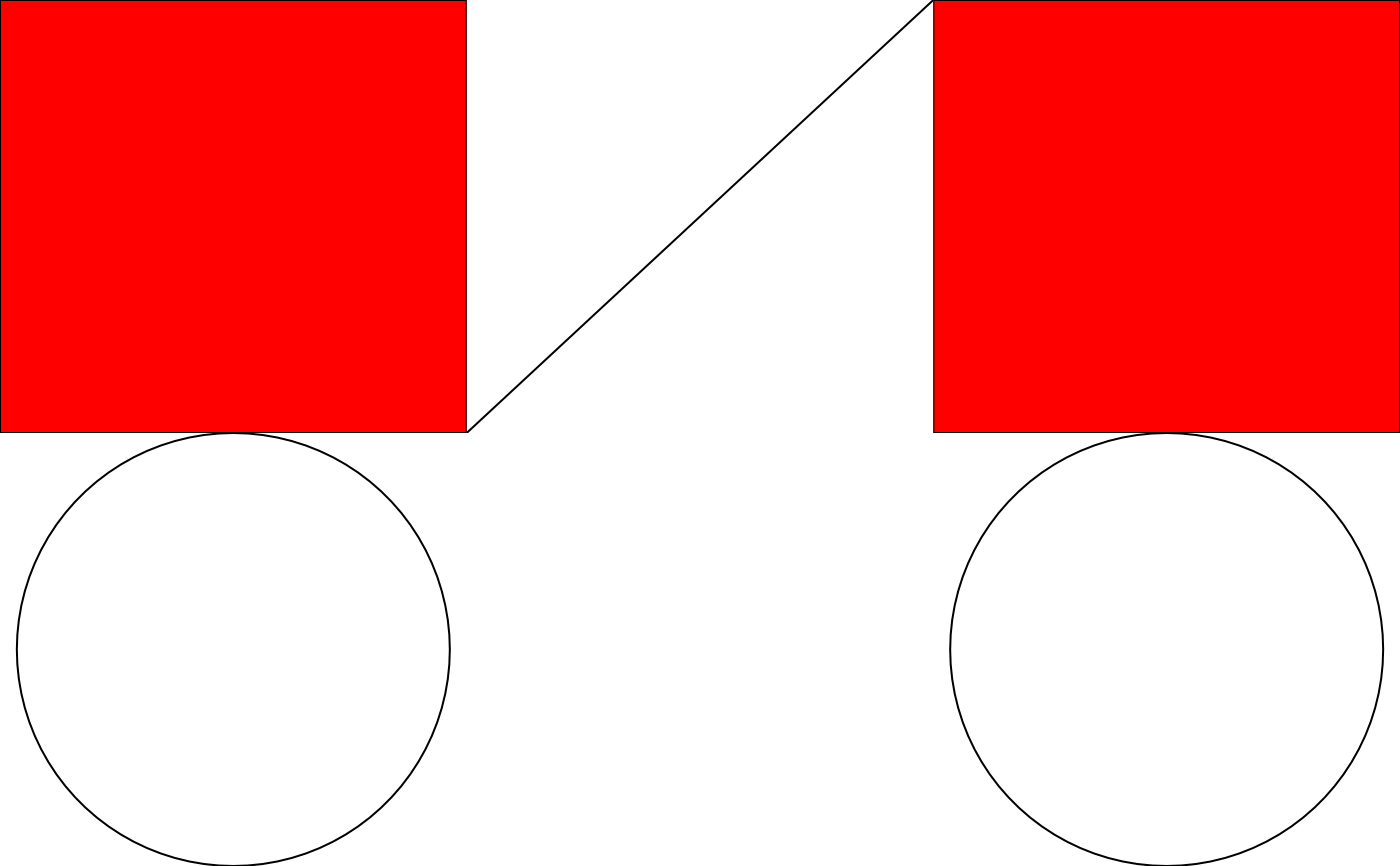These functions are the parallels of the matrix/data.frame row and column bindings. As such they work in the same way, except they have to take care of additional attributes within the gtables. Most importantly it needs to take care of the sizing of the final gtable, as the different gtables going in may have different widths or heights. By default it tries to calculate the maximum width/height among the supplied gtables, but other options exists. Further, the relative layering of the grobs in each gtable can be modified or left as-is.

# S3 method for gtable
rbind(..., size = "max", z = NULL)

# S3 method for gtable
cbind(..., size = "max", z = NULL)

## Arguments

... gtables to combine (x and y) How should the widths (for rbind) and the heights (for cbind) be combined across the gtables: take values from first, or last gtable, or compute the min or max values. Defaults to max. A numeric vector indicating the relative z values of each gtable. The z values of each object in the resulting gtable will be modified to fit this order. If NULL, then the z values of obects within each gtable will not be modified.

A gtable object

## Examples

library(grid)
a <- rectGrob(gp = gpar(fill = "red"))
b <- circleGrob()
c <- linesGrob()

row <- matrix(list(a, b), nrow = 1)
col <- matrix(list(a, b), ncol = 1)
mat <- matrix(list(a, b, c, nullGrob()), nrow = 2)

row_gt <- gtable_matrix("demo", row, unit(c(1, 1), "null"), unit(1, "null"))
col_gt <- gtable_matrix("demo", col, unit(1, "null"), unit(c(1, 1), "null"))
mat_gt <- gtable_matrix("demo", mat, unit(c(1, 1), "null"), unit(c(1, 1), "null"))

# cbind
c_binded <- cbind(mat_gt, col_gt, size = "first")
plot(c_binded)# rbind
r_binded <- rbind(mat_gt, row_gt, size = "last")
plot(r_binded)# Dimensions must match along bind direction
try(cbind(mat_gt, row_gt))#> Error : x and y must have the same number of rows